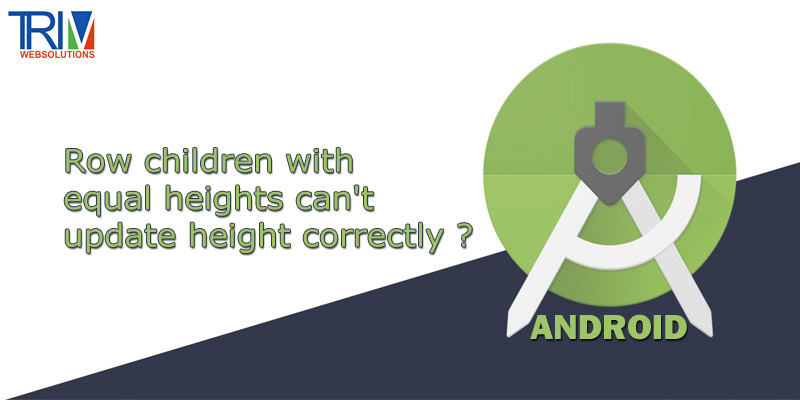Quick Inquiry

# Row children with equal heights can't update height correctly in android ?Try This

``` ```@Composable
fun IssueWithRowHeight() {
var longerText by remember {
mutableStateOf("")
}
LaunchedEffect(key1 = true) {
delay(2000)
longerText =
"this is long text this is long text this is long text this is long text this is long text"
}

//If you use this and comment the launched effect the row has the correct height
//    var longerText by remember {
//        mutableStateOf("this is long text this is long text this is long text this is long text this is long text")
//    }

Column {
Row(
modifier = Modifier
.fillMaxWidth()
.wrapContentHeight(),
) {
Row(
modifier = Modifier
.weight(1f)
.clickable {  }
.padding(PaddingValues(start = 16.dp, top = 8.dp, end = 16.dp, bottom = 8.dp))
) {
Text(text = "short text")
}
Row(
modifier = Modifier
.weight(1f)
.clickable {  }
.padding(PaddingValues(start = 16.dp, top = 8.dp, end = 16.dp, bottom = 8.dp))
) {
Text(text = longerText)
}
}

//Just for reference that the string is actually updating..
Row(
modifier = Modifier
.fillMaxWidth()
.background(Color.Red)
) {
Text(text = longerText)
}
}
}``````